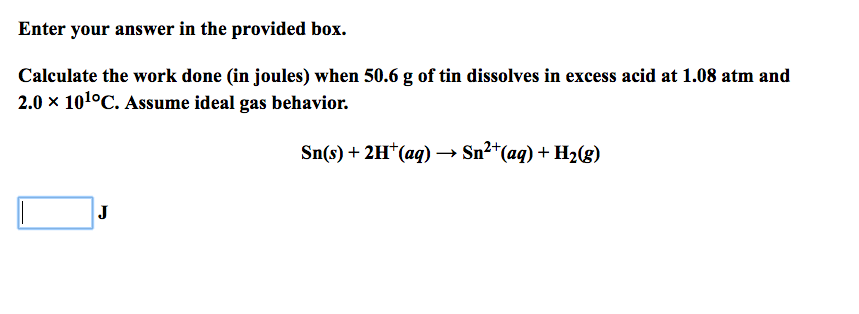# Enter your answer in the provided box. Calculate the work done (in joules) when 50.6 g of tin dissolves in

###### Question:Enter your answer in the provided box. Calculate the work done (in joules) when 50.6 g of tin dissolves in excess acid at 1.08 atm and 2.0 x 101°C. Assume ideal gas behavior. Sn(s) + 2H+ (aq) → Sn2+(aq) + H2(g)
Enter your answer in the provided box. Calculate the work done (in joules) when 50.6 g of tin dissolves in excess acid at 1.08 atm and 2.0 x 101°C. Assume ideal gas behavior. Sn(s) + 2H+ (aq) → Sn2+(aq) + H2(g)

#### Similar Solved Questions

##### Which of the following about the article and the graph is correct?
which of the following about the article and the graph is correct?...
##### Tatum Company has four products in its inventory. Information about the December 31, 2021, inventory is...
Tatum Company has four products in its inventory. Information about the December 31, 2021, inventory is as follows: Product 101 102 Total cost $122,000 91,000 61,000 31,000 Total Net Realizable Value$101,000 111,000 51,000 51,000 Required: 1. Determine the carrying value of inventory at December 31...